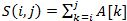# GATE | GATE CS 2019 | Question 34

• Last Updated : 19 Feb, 2019

Consider a sequence of 14 elements: A = [-5, -10, 6, 3, -1, -2, 13, 4, -9, -1, 4, 12, -3, 0]. The subsequence sum. Determine the maximum of S(i,j), where 0 ≤ i ≤ j < 14. (Divide and conquer approach may be used)
Note: This was Numerical Type question.
(A) 29
(B) 19
(C) 39
(D) 09

Explanation: According to largest Sum Contiguous subarray is from index 2 to 11,

```Max (S(i, j))
= S(2, 11)
= 6 + 3 + (-1) + (-2) + 13 + 4 + (-9) + (-1) + 4 + 12
= 29 ```

So, answer is 29. See: https://ide.geeksforgeeks.org/NR2BbxpD7j

Quiz of this Question

My Personal Notes arrow_drop_up